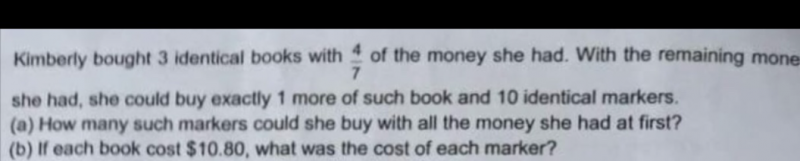# QuestionHi pls help me with this question. Thks

This is a Simultaneous Equation question.

1. Buy 1. 4u money buy 3 books.

2. Buy 2. 3u money buy 1 book and 10 marker.

 Buy 1 buy 2 buy 2 x 3 B2 – B1 Book 3 1 3 0 Marker 10 30 30 Total 4u 3u 9u 5u

5u = 30 marker

1u = 30 ÷ 5 = 6

a)

7u = 6 × 7 = 42 marker

b)

3 book = 3 × 10.8 = 32.8

4u = 32.4

1u = 32.4 ÷ 4 = 8.1

7u = 8.1 × 7 = 56.7

56.7 ÷ 42 =\$1.35

0 Replies 0 Likes

4/7 can’t divide into 3 units nicely. So let’s look at it as 12/21 instead.
We can use units and parts to solve this from here,

Let what Kim had at first be 21u
3 books = 12u, then each book will be 4u.

Of the remaining money= 21u – 12u = 9u
10 markers = 9u – 4u = 5u

a) one marker = 5u/10 = 0.5u
She could have bought = 21u/0.5u = 42 markers##

b) 4u = \$10.80
u = 10.80/4 = \$2.70
Marker = 0.5u = 2.7/2 = \$1.35#

0 Replies 0 Likes